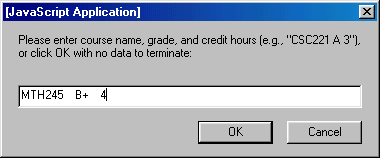### CSC 551: Web Programming Fall 2001 HW3: JavaScript Programming

Create a Web page named gpa.html that can be used to compute your grade point average given grades and hours for courses completed. The page should repeatedly prompt the user for course information, reading the course name, grade received, and credit hours for a particular course in a single dialog box. The program should continue prompting and reading in grades until the user enters an empty string to signify the end. For example,Once the course grades have been stored, the page should compute the student's overall GPA. According to the Creighton University Bulletin, grade point averages are computed as follows:

1. Grade points (a.k.a. quality points) are assigned to each course based on the grade and number of credit hours:
• A yields 4 points for each hour
• B+ yields 3.5 points for each hour
• B yields 3 points for each hour
• C+ yields 2.5 points for each hour
• C yields 2 points for each hour
• D yields 1 point for each hour
• F, AF, and WF yield 0 points for each hour
2. The grade point average (a.k.a. quality point average) is computed by dividing the total number of grade points earned by the total number of credit hours.

For example, suppose a student entered the following course information (with each line entered in a different dialog box):

THL100 B+ 3 PHL107 A 3 ENG120 C+ 3 MTH245 A 4 CSC221 A 3

The total number of grade points earned by this student is

(3.5 * 3) + (4 * 3) + (2.5 * 3) + (4 * 4) + (4 * 3) = 10.5 + 12 + 7.5 + 16 + 12 = 58

Dividing this total by the number of credit hours completed yields a GPA of 58/16 = 3.625.

For 90% credit: your page should display the total number of grade points, credit hours, and GPA for the student. For example,

 ``` Total grade points = 58 Number of hours = 16 GPA = 3.625 ```

For 100% credit: your page should also display all of the course information in a readable form. This information should not be displayed in the page until all user input has been processed. For example,

 ``` COURSE GRADE HOURS THL100 B+ 3 PHL107 A 3 ENG120 C+ 3 MTH245 A 4 CSC221 A 3 Total grade points = 58 Number of hours = 16 GPA = 3.625 ```

Hint: For processing the information on each course, you should utilize the Arrayify function defined in the lectures. This function will allow you to take the string returned by the prompt function and extract the three pieces of data (name, grade, and hours). Note that the Arrayify function stores each piece of data as a string, so you must use the parseFloat function to convert the credit hours into a number.